# High School Math : Identifying Variables and Relationships

## Example Questions

### Example Question #1 : Independent Random Variable Combination

In a standard deck of cards, with replacement, what is the probability of drawing two Ace of Hearts?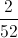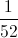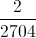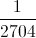Explanation:

Probability of drawing first ace of hearts: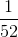Probability of drawing second ace of hearts:Multiply both probabilities by each other: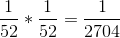### Example Question #10 : How To Use The Multiplication Rule

In a standard deck of cards, without replacement, what is the probability of drawing three kings?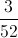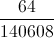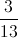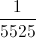Explanation:

Start with 52 cards, probability of drawing first king: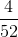Now you have 51 cards. Probability of drawing second king: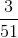Now you have 50 cards. Probability of drawing third king: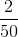Multiply all probabilities: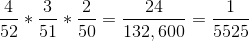### Example Question #1 : Variable Relationships

With a standard deck of cards, what is the probability of picking a spade then a red card if there is no replacement?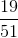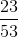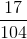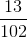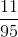Explanation:

In a standard deck of cards: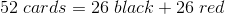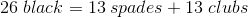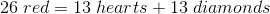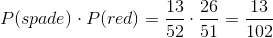### Example Question #68 : Ap Statistics

Using a standard deck of cards, what is the probability of choosing a single face card?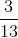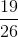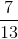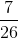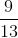There arecards in a standard deck:cards insuits.  There areface cards (King, Queen, Jack) in each suit, so there aretotal face cards.
Thus the probability of choosing a single face card is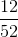or.